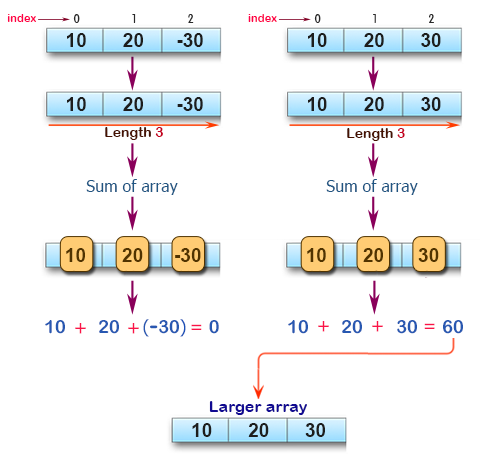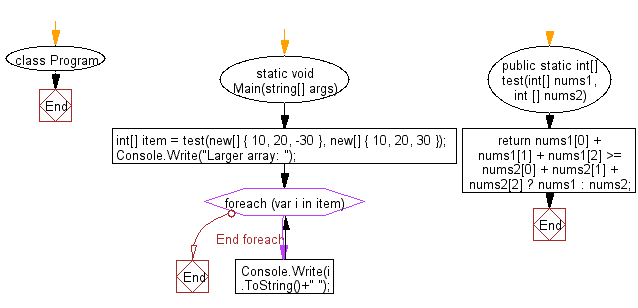﻿ C# - Largest sum of the two arrays of integers, length 3# C# Sharp Basic Algorithm Exercises: Compute the sum of the two given arrays of integers, length 3 and find the array which has the largest sum

## C# Sharp Basic Algorithm: Exercise-102 with Solution

Write a C# Sharp program to compute the sum of the two given arrays of integers, length 3 and find the array which has the largest sum.

Pictorial Presentation:Sample Solution:-

C# Sharp Code:

``````using System;
namespace exercises
{
class Program
{
static void Main(string[] args)
{
int[] item =  test(new[] { 10, 20, -30 }, new[] { 10, 20, 30 });
Console.Write("Larger array: ");
foreach(var i in item)
{
Console.Write(i.ToString()+" ");
}
}
public static int[]  test(int[] nums1, int [] nums2)
{
return nums1 + nums1 + nums1 >= nums2 + nums2 + nums2 ? nums1 : nums2;
}
}
}
```
```

Sample Output:

`Larger array: 10 20 30`

Flowchart:C# Sharp Code Editor:

Improve this sample solution and post your code through Disqus

What is the difficulty level of this exercise?

Test your Programming skills with w3resource's quiz.

﻿